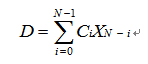## 单片机数字滤波的算法

单片机主要作用是控制外围的器件，并实现一定的通信和数据处理。但在某些特定场合，不可避免地要用到数学运算，尽管单片机并不擅长实现算法和进行复杂的运算。下面主要是介绍如何用单片机实现数字滤波。

l         数字滤波无需其他的硬件成本，只用一个计算过程，可靠性高，不存在阻抗匹配问题。尤其是数字滤波可以对频率很低的信号进行滤波，这是模拟滤波器做不到的。
l         数字滤波使用软件算法实现，多输入通道可共用一个滤波程序，降低系统开支。
l         只要适当改变滤波器的滤波程序或运算，就能方便地改变其滤波特性，这对于滤除低频干扰和随机信号会有较大的效果。
l         在单片机系统中常用的滤波算法有限幅滤波法、中值滤波法、算术平均滤波法、加权平均滤波法、滑动平均滤波等。

（1）限幅滤波算法

" title="cccccc">">#cccccc">#define A                //允许的最大差值
char data;                //上一次的数据
char filter()
{
char datanew;        //新数据变量
datanew=get_data();   //获得新数据变量
if( (datanew-data)>A||(data-datanew>A) )
return data;
else
return datanew;
}

（2）中值滤波算法

" title="cccccc">">#cccccc">#define N 11    //定义获得的数据个数
char filter()
{
char value_buff[N];            //定义存储数据的数组
char count,i,j,temp;
for(count=0;count<N;count++) //获取数据
{
value_buf[count]=get_data();
delay();                 //如果采集数据比较慢，那么就需要延时或中断
}

for(j=0;j<N-1;j++)            //用冒泡法对数据进行排序，当然最好用其他排序方法
{
for(value_buff[i]>value_buff[i+1]
{
temp=value_buff[i];
value_buff[i]=value_buff[i+1];
value_buff[i+1]=temp;
}
}
return value_buff[(N-1)/2];
}

(3)算术平均滤波算法

char filter()
{
int sum=0;
for (count=0;count<N;count++)
{
sum+=get_data();
delay():
}
return (char)(sum/N);
}

（4）加权平均滤波算法char code jq[N]={1,2,3,4,5,6,7,8,9,10,11,12}; //code数组为加权系数表，存在程序存储区
char code sum_jq=1+2+3+4+5+6+7+8+9+10+11+12;
char filter()
{
char count;
char value_buff[N];
int sum=0;
for(count=0;count<N;count++)
{
value_buff[count]=get_data();
delay();
}
for(count=0;count<N;count++)
sum+=value_buff[count]*jq[count];
return (char)(sum/sum_jq);
}

（5）滑动平均滤波算法

char value_buff[N];
char i=0;
char filter()
{
char count;
int sum=0;
value_buff[i++]=get_data();
if(i==N)
i=0;
for(count=0;count<N;count++)
sum=value_buff[count];
return (char)(sum/N);
}